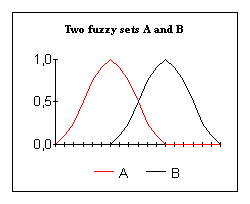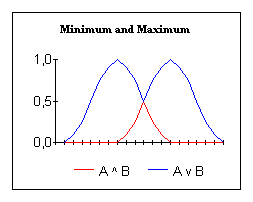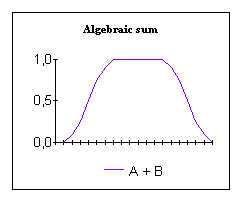# T-norms and S-norms

## Important set connectives

For better reading I write for μA(x) (= the grade of membership of x in A): A.
So A is the fuzzy set A.
Now let's take two fuzzy sets A and B.The minimum operator is the well known operator to model intersection. It is the red line in the picture below.This minimum operator is one of the "triangular norms".
With the T-norms there are the T-conorms, also called the S-norms.
They model union. The maximum operator is an S-norm.
It is the blue line in the picture above.
Apart from that I mention the algebraic sum of A and B: A + BT-norms and S-norms are logical duals and can be computed from each other by:

### A few well known couples of T-norms and S-norms

 twsw Drastic productDrastic sum tw(A,B) = min (A,B) if max (A,B) = 1, else = 0sw(A,B) = max (A,B) if min (A,B) = 0, else = 1 t1s1 Bounded differenceBounded sum t1(A,B) = max (0, A + B - 1)s1(A,B) = min (1, A + B) t1.5s1.5 Einstein productEinstein sum t1.5(A,B) = (AB/(2 - [ A + B - AB])s1.5(A,B) = (A + B)/ (1 + AB) t2s2 Algebraic productProbabilistic sum t2(A,B) = ABs2(A,B) = A + B - AB t2.5s2.5 Hamacher productHamacher sum t2.5(A,B) = (AB)/(A + B - AB)s2.5(A,B) = (A + B - 2AB)/(1 - AB) t3s3 MinimumMaximum t3(A,B) = min (A,B)s3(A,B) = max (A,B)

They have the following relationship:

 tw <= t1 <= t1.5 <= t2 <= t2.5 <= t3 s3 <= s2.5 <= s2 <= s1.5 <= s1 <= sw

### Operators that are more general

 Symbole Name Formula FAND Fuzzy AND FAND(A,B) = γ*min(A,B) + 0.5(1-γ)(A + B)    γ Î [0,1] FOR Fuzzy OR FOR(A,B) = γ*max(A,B) + 0.5(1-γ)(A + B)      γ Î [0,1]

Even more general are convex combinations of T-norms with their S-norms. For example the convex combination of the minimum and the maximum operator. Or of the algebraic product and the probabilistic sum.

### Some convex combinations of T-norms with their S-norms

 Name Formula Convex combination of min and max Comb(A,B)= γ*min(A,B)+(1-γ)*max(A,B)    γ Î [0,1] Convex combination of algebraic product and probabilistic sum Comb(A,B)= γ*(AB)+(1- γ)*(A+B-AB)       γ Î [0,1]

So it's obvious that there are a lot of operators to model union and intersection.
This is one of the features of fuzzy logic, that makes it so well suited to handel the vagueness of the real world.

Source: T.Tilly, "FUZZY LOGIC, theorie, praktijk, hard-en software", Kluwer Techniek, ELEKTRO/ELEKTRONICA.

Back: Fuzzy Sets

Home: Homepage

Last edited: